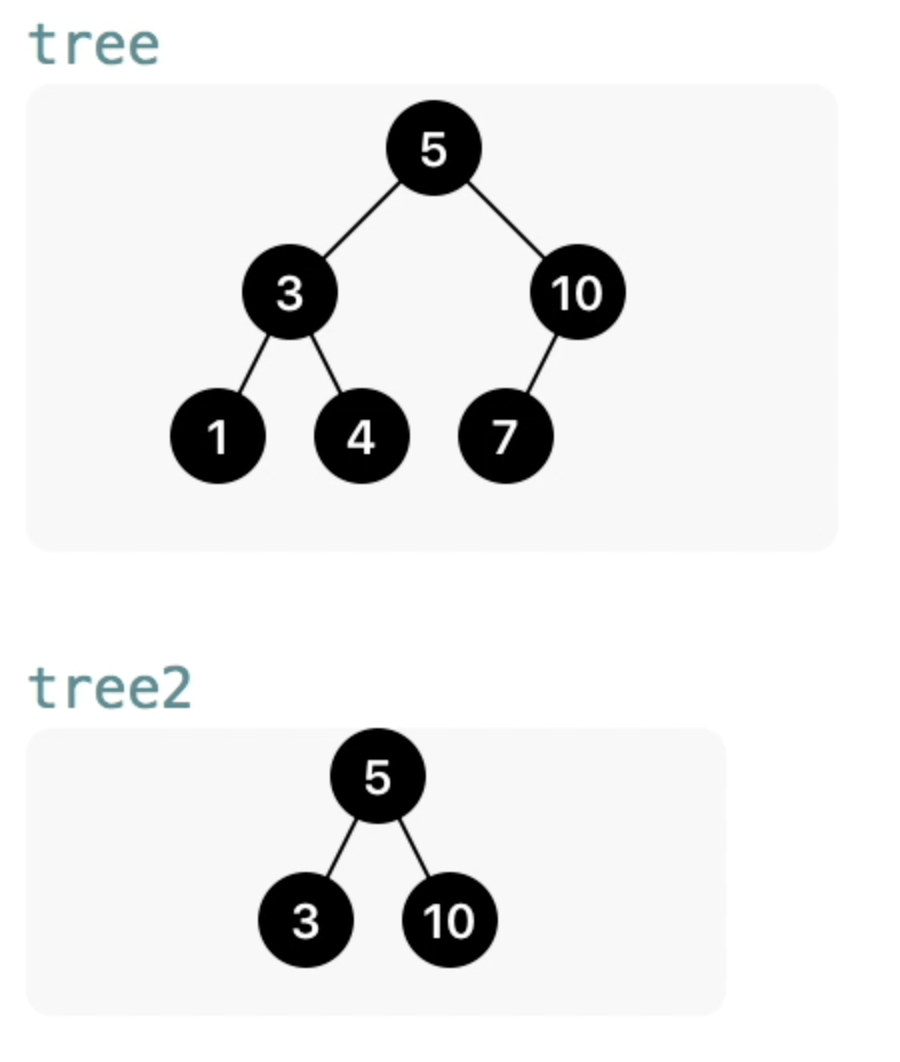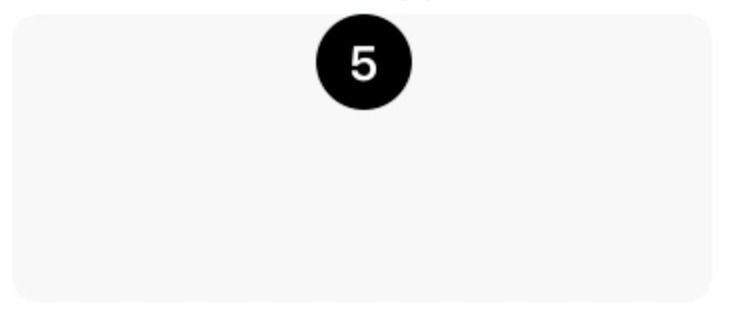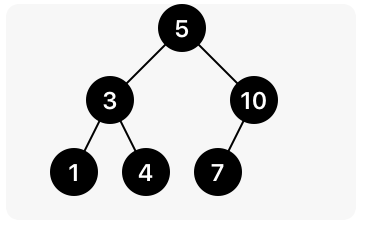Swift Talk # 65

## Playground QuickLook for Binary Trees

### This episode is freely available thanks to the support of our subscribers

We create a custom Quick Look extension to visualize binary tree structures in playgrounds.

00:06 In episode #58, together with Károly, we implemented red-black trees just as they're done in his book Optimizing Collections. To visualize the trees, we rendered them in the playground. Today we'll take a closer look at how we did this by implementing Quick Look for binary trees.

00:40 We look at two example trees: one with three levels, and another one with two levels. We'll use the number of levels — the height of the tree — to figure out the bounds of the image we want to draw. On the bottom row, we can draw all nodes next to each other. One level up, there's more spacing between the nodes such that they're each centered above their two children, and so on and so forth. This means that the horizontal spacing between nodes is a function of the height:## Computing the Tree's Height

02:17 The first step in creating an image from a tree is to compute the height of the tree. Then we can use this height to determine the horizontal positioning of nodes as we work our way down the tree.

02:48 We'll extend the `BinaryTree` implementation from episode #56 to render an image. We compute the height of the tree by recursively going down all its paths, and we switch over the tree enum and return zero for empty nodes. For non-empty nodes, we return the height of the longest subtree, incremented by one:

``````extension BinaryTree {
var maxHeight: Int {
switch self {
case .empty: return 0
case let .node(_, left, right):
return max(left.maxHeight, right.maxHeight) + 1
}
}
}
``````

## Computing the Bounds

05:07 From this height, we can compute the bounds of the entire image. The height of the tree — sometimes referred to as the depth — gives us the maximum number of nodes on the bottom row. Because each node can have two children, the maximum number of nodes at the bottom of a tree equals two to the power of the depth of the tree.

06:04 We add a `bounds` property, which is a `CGRect`. The width is the maximum number of nodes at the bottom, multiplied by a constant node size (later we'll add some spacing between nodes):

``````let nodeSize = CGSize(width: 24, height: 24)

extension BinaryTree {
// ...
var bounds: CGRect {
let width = pow(2, CGFloat(maxHeight)-1) * nodeSize.width
let height = CGFloat(maxHeight) * nodeSize.height
return CGRect(origin: .zero, size: CGSize(width: width, height: height))
}
}
``````

## Rendering the Tree

08:03 Next, we can start writing the `render` method using the bounds and `UIGraphicsImageRenderer`. We write a helper method that, given the center `CGPoint` of a node and the current level, draws that node into the context of the renderer:

``````func render(into context: CGContext, at center: CGPoint, currentHeight: Int) {
// TODO
}

func render() -> UIImage {
let center = CGPoint(x: bounds.midX, y: nodeSize.height/2)
return UIGraphicsImageRenderer(bounds: bounds).image() { context in
self.render(into: context.cgContext, at: center, currentHeight: self.maxHeight)
}
}
``````

11:34 In the `render(into:)` method, we first deal with the empty case again. If the tree is empty, we simply return. In the same statement, we can get out the element and the subtrees:

``````func render(into context: CGContext, at center: CGPoint, currentHeight: Int) {
guard case let .node(element, left, right) = self else { return }
// TODO
}
``````

12:39 When we know that we have an element, we can draw the node. We use the `fillEllipse` helper method, which only needs a center point and a size. And for the label, we draw the element as a string over the node using some string attributes we've defined elsewhere:

``````func render(into context: CGContext, at center: CGPoint, currentHeight: Int) {
guard case let .node(element, left, right) = self else { return }

UIColor.black.setFill()
context.fillEllipse(in: CGRect(center: center, size: nodeSize))
"\(element)".draw(center: center, attributes: nodeAttributes)
}
``````

14:21 We can already see an image at this point:

``````tree.render()
``````## Recursive Rendering

14:44 We only see the top node of the tree since we're not yet drawing the subtrees. We'll do so by recursively calling the same `render(into:)` method for both the left and right subtrees. For this, we need to calculate their center points based on the current center point and an offset. The vertical offset is the constant node height, and we calculate the horizontal offset from `currentHeight`:

``````let offset = // TODO
if case .node = left {
let leftCenter = CGPoint(x: center.x-offset, y: center.y+nodeSize.height)
left.render(into: context, at: leftCenter, currentHeight: currentHeight-1)
}
``````

16:49 From `currentHeight` we calculate how many nodes can fit on the current row, just like before. This gives us the total width of the row, which we divide by two to get the offset between the current center and the subtree's center:

``````let offset = pow(2, CGFloat(currentHeight-1) * nodeSize.width / 2
``````

18:14 Something is still off, because in the `render` method we started rendering nodes with the wrong `currentHeight`. Maybe it wasn't so smart to not use a zero-based height after all. When we subtract 1 we fix the issue, and now we see the entire left side of the tree being drawn. We copy the code and update it to draw the right subtree as well:

``````if case .node = right {
let rightCenter = CGPoint(x: center.x+offset, y: center.y+nodeSize.height)
right.render(into: context, at: rightCenter, currentHeight: currentHeight-1)
}
``````

19:30 We can easily add lines between the nodes using the center points of the current node and the left and right subtrees. It's important that we draw the lines first, the nodes next, and the labels on top of everything last. The complete method now looks like this:

``````func render(into context: CGContext, at center: CGPoint, currentHeight: Int) {
guard case let .node(element, left, right) = self else { return }

let offset = pow(2, CGFloat(currentHeight-1) * nodeSize.width / 2
if case .node = left {
let leftCenter = CGPoint(x: center.x-offset, y: center.y+nodeSize.height)
context.drawLine(from: center, to: leftCenter)
left.render(into: context, at: leftCenter, currentHeight: currentHeight-1)
}
if case .node = right {
let rightCenter = CGPoint(x: center.x+offset, y: center.y+nodeSize.height)
context.drawLine(from: center, to: rightCenter)
right.render(into: context, at: rightCenter, currentHeight: currentHeight-1)
}

UIColor.black.setFill()
context.fillEllipse(in: CGRect(center: center, size: nodeSize))
"\(element)".draw(center: center, attributes: nodeAttributes)
}
``````

20:37 We can clean this up a bit by using a local function instead of having almost duplicate code to draw both subtrees:

``````func render(into context: CGContext, at center: CGPoint, currentHeight: Int) {
guard case let .node(element, left, right) = self else { return }

let offset = pow(2, CGFloat(currentHeight)-1) * nodeSize.width
func recurse(child: BinaryTree, offset: CGFloat) {
guard case .node = child else { return }
let childCenter = CGPoint(x: center.x+offset, y: center.y+nodeSize.height)
context.drawLine(from: center, to: childCenter)
child.render(into: context, at: childCenter, currentHeight: currentHeight-1)

}
recurse(child: left, offset: -offset)
recurse(child: right, offset: offset)

UIColor.black.setFill()
context.fillEllipse(in: CGRect(center: center, size: nodeSize))
"\(element)".draw(center: center, attributes: nodeAttributes)
}
``````

23:03 Everything is drawn very tightly now, but it'd be nice to add some spacing between the nodes. We define a tuple with factors for the node width and height:

``````let spacing = (horizontal: 1.5 as CGFloat, vertical: 1.5 as CGFloat)
``````

24:04 We use this spacing in our calculation of the bounds. We ignore the fact that this gives us some extra space — not just between nodes, but also outside the tree:

``````var bounds: CGRect {
let width = pow(2, CGFloat(maxHeight)-1) * nodeSize.width * spacing.horizontal
let height = CGFloat(maxHeight) * nodeSize.height * spacing.vertical
return CGRect(origin: .zero, size: CGSize(width: width, height: height))
}
``````

24:54 We also have to apply the spacing factor to the offset between nodes:

``````func render(into context: CGContext, at center: CGPoint, currentHeight: Int) {
// ...
let offset = pow(2, CGFloat(currentHeight)-1) * nodeSize.width * spacing.horizontal / 2

func recurse(child: BinaryTree, offset: CGFloat) {
// ...
let childCenter = CGPoint(x: center.x+offset, y: center.y + nodeSize.height*spacing.vertical)
// ...

}
// ...
}
``````

25:24 With this in place, we render a nice-looking image:## Quick Look

25:35 Finally, we can implement Quick Look for `BinaryTree` so that we can view the image representation of a tree without manually calling the render method:

``````extension BinaryTree: CustomPlaygroundQuickLookable {
public var customPlaygroundQuickLook: PlaygroundQuickLook {
return .image(render())
}
}
``````

26:52 Another cool improvement would be to animate nodes and branches when the tree updates, but that would probably require an entirely different implementation using subviews and constraints.

27:12 It's worth noting we didn't write this code in half an hour. It took us quite a bit of time, with three developers, to figure out the math and then to simplify the implementation.

### Resources

• #### Playground

Written in Swift 4

See All
SwiftUI

### Interactive Marquee View (Part 1)

Episode 374 · Sep 29

SwiftUI

### Cubic Bezier Keyframes (Part 5)

Episode 373 · Sep 22

SwiftUI

### Cubic Bezier Keyframes (Part 4)

Episode 372 · Sep 15

SwiftUI

### Cubic Bezier Keyframes (Part 3)

Episode 371 · Sep 08

SwiftUI

### Cubic Bézier Keyframes (Part 2)

Episode 370 · Sep 01

SwiftUI

### Cubic Bézier Keyframes (Part 1)

Episode 369 · Aug 25

SwiftUI

### Building Keyframe Animations (Part 3)

Episode 368 · Aug 18

SwiftUI

### Building Keyframe Animations (Part 2)

Episode 367 · Aug 11

Unlock Full Access

## Subscribe to Swift Talk

• ### Watch All Episodes

A new episode every week